##### Geometry Essentials For Dummies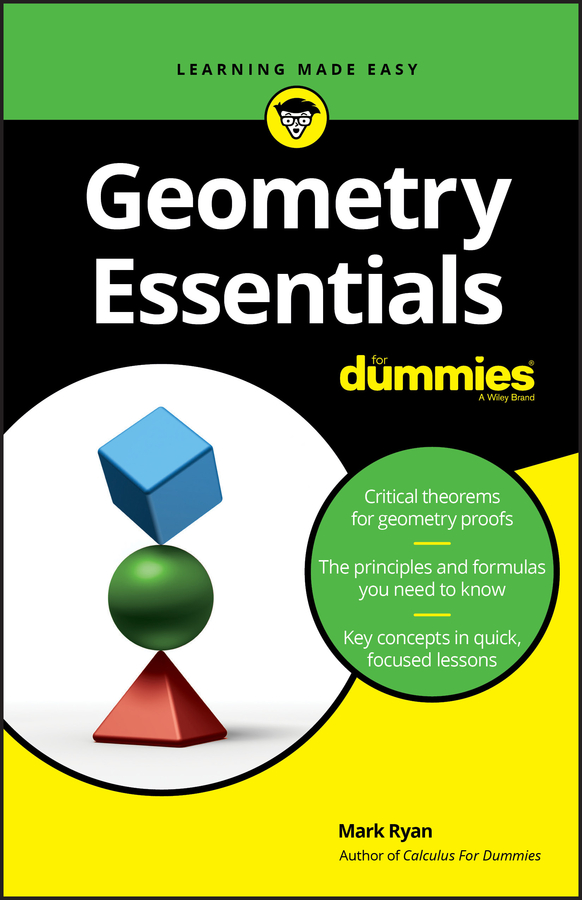The triangle inequality principle states that the sum of the lengths of any two sides of a triangle must be greater than the length of the third side. This idea comes up in a fair number of problems, so don’t forget it! It’s based on the simple fact that the shortest distance between two points is a straight line. Check out the following figure and the explanation that follows.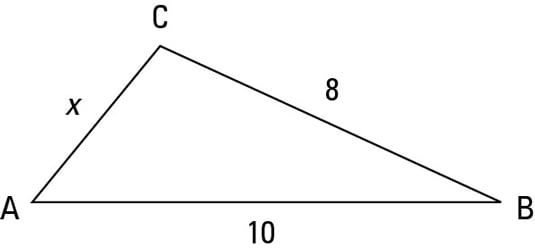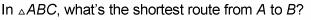Naturally, going straight across from A to B is shorter than taking a detour by traveling from A to C and then on to B. That’s the triangle inequality principle in a nutshell.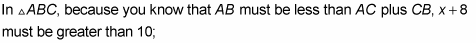therefore,

x + 8 > 10

x > 2

But don’t forget that the same principle applies to the path from A to C; thus, 8 + 10 must be greater than x:

8 + 10 > x

18 > x

You can write both of these answers as a single inequality:

2 < x < 18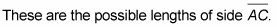The following figure shows this range of lengths. Think of vertex B as a hinge. As the hinge opens more and more, the length of segment AC grows.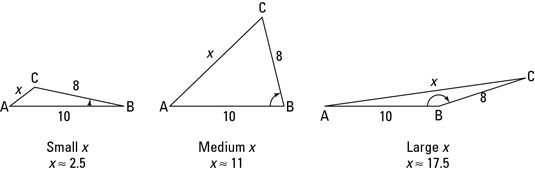Note: Give yourself a pat on the back if you’re wondering why the third path wasn’t mentioned, from B to C. Here’s why: In the first two inequalities, note that the longer known side (the 10) and the unknown side (the x) were put on the right sides of the inequalities. That’s all you need to do to get your answer. You don’t have to do a third inequality with the shorter of the two known sides (the 8) on the right side of the inequality, because that won’t add anything to your answer.

By the way, if this problem had been about three towns A, B, and C instead of triangle ABC, then the possible distances between towns A and C would look the same except that the less-than symbols would be less-than-or-equal-to symbols: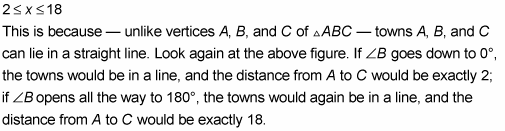You can’t do this with the triangle problem, however, because when A, B, and C are in a line, there’s no triangle left.